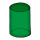# System of equations + bikvadratic equation - math problems

#### Number of problems found: 10

• Hyperbola equationFind the hyperbola equation with the center of S [0; 0], passing through the points: A [5; 3] B [8; -10]
• Right triangle eq2Find the lengths of the sides and the angles in the right triangle. Given area S = 210 and perimeter o = 70.
• Rotary cylinderIn the rotary cylinder it is given: surface S = 96 cm2 and volume V = 192 cm cubic. Calculate its radius and height.
• Sequences AP + GPThe three numbers that make up the arithmetic sequence have the sum of 30. If we subtract from the first 5, from the second 4 and keep the third, we get the geometric sequence. Find AP and GP members.
• The cylinderIn a rotating cylinder it is given: the surface of the shell (without bases) S = 96 cm2 and the volume V = 192 cm cubic. Calculate the radius and height of this cylinder.
• CuboidCuboid with edge a=6 cm and space diagonal u=31 cm has volume V=900 cm3. Calculate the length of the other edges.
• Faces diagonalsIf a cuboid's diagonals are x, y, and z (wall diagonals or three faces), then find the cuboid volume. Solve for x=1.3, y=1, z=1.2
• Two chordsCalculate the length of chord AB and perpendicular chord BC to circle if AB is 4 cm from the center of the circle and BC 8 cm from the center of the circle.
• MO Z8-I-1 2018Fero and David meet daily in the elevator. One morning they found that if they multiply their current age, they get 238. If they did the same after four years, this product would be 378. Determine the sum of the current ages of Fero and David.
• Eq2 2Solve following equation with quadratic members and rational function: (x2+1)/(x-4) + (x2-1)/(x+3) = 23

We apologize, but in this category are not a lot of examples.
Do you have an interesting mathematical word problem that you can't solve it? Submit a math problem, and we can try to solve it.

We will send a solution to your e-mail address. Solved examples are also published here. Please enter the e-mail correctly and check whether you don't have a full mailbox.

Please do not submit problems from current active competitions such as Mathematical Olympiad, correspondence seminars etc...

Do you have a system of equations and looking for calculator system of linear equations? System of equations - math word problems. Bikvadratic equation - math word problems.